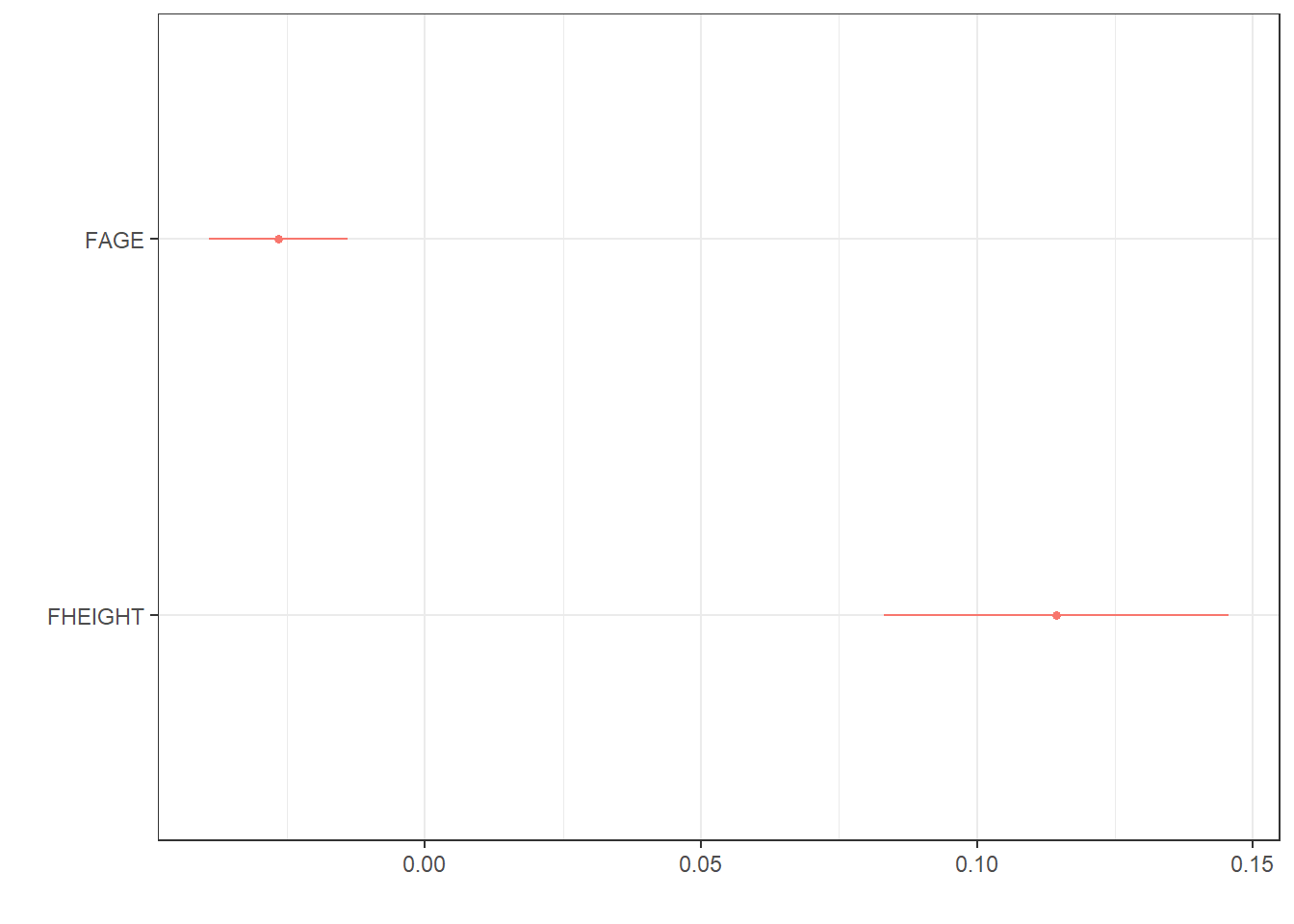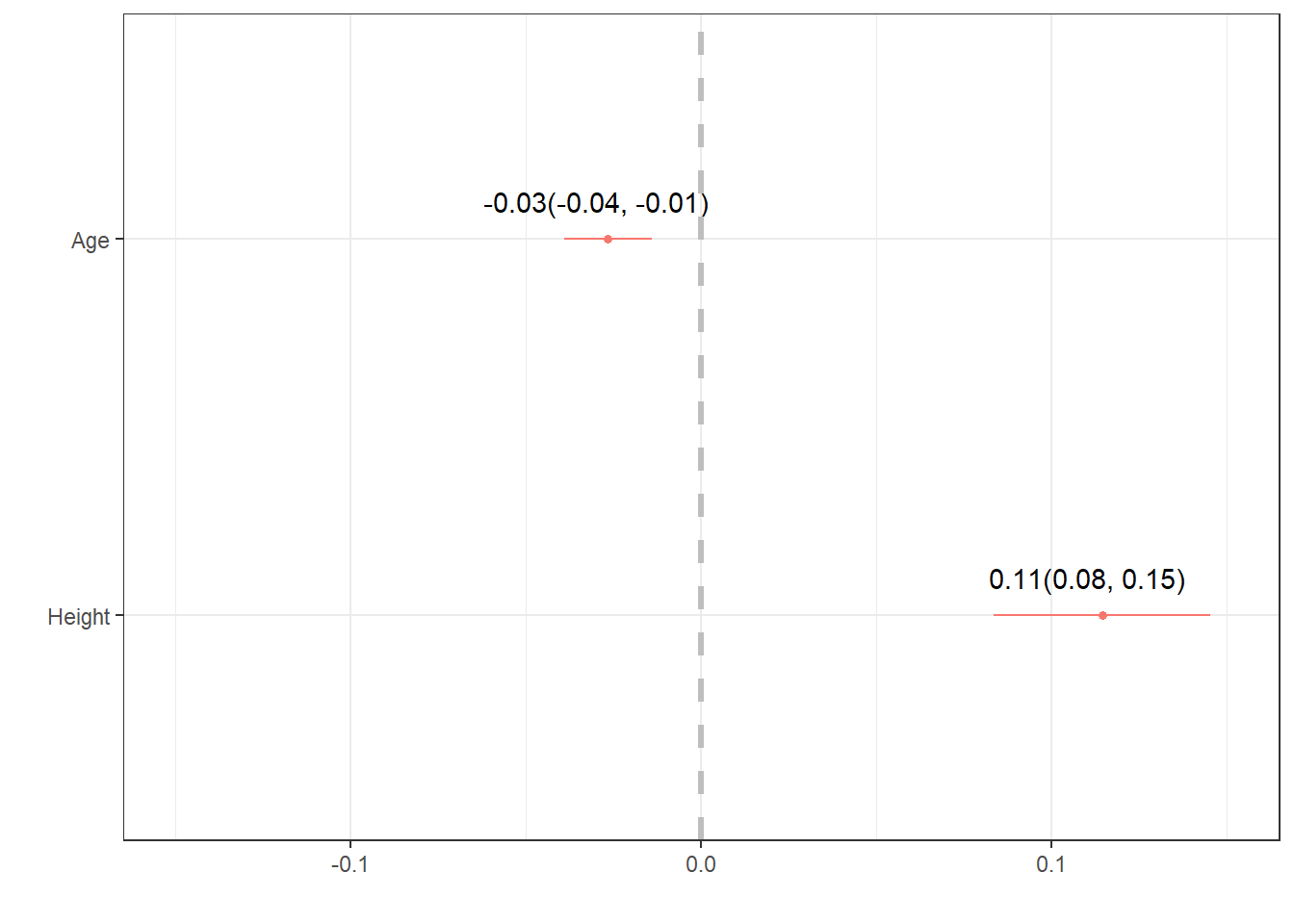## 9.5 Presenting results

The direct software output always tells you more information than what you are wanting to share with an audience. Here are some ways to “prettify” your regression output.

• Using tidy and kable
library(broom)
tidy(mlr.dad.model) |> kable(digits=3)
term estimate std.error statistic p.value
(Intercept) -2.761 1.138 -2.427 0.016
FAGE -0.027 0.006 -4.183 0.000
FHEIGHT 0.114 0.016 7.245 0.000
library(gtsummary)
tbl_regression(mlr.dad.model)
Characteristic Beta 95% CI1 p-value
FAGE -0.03 -0.04, -0.01 <0.001
FHEIGHT 0.11 0.08, 0.15 <0.001
1 CI = Confidence Interval
library(dotwhisker)
dwplot(mlr.dad.model)• Improvement on dwplot - extract the point estimate & CI into a data table, then add it as a geom_text layer.
text <- data.frame(                               # create a data frame
estimate = coef(mlr.dad.model),                 # by extracting the coefficients,
CI.low = confint(mlr.dad.model)[,1],            # with their lower
CI.high = confint(mlr.dad.model)[,2]) %>%       # and upper confidence interval values
round(2)                                        # round digits

# create the string for the label
text$label <- paste0(text$estimate, "(", text$CI.low, ", " , text$CI.high, ")")

text                                              # view the results to check for correctness
##             estimate CI.low CI.high               label
## (Intercept)    -2.76  -5.01   -0.51 -2.76(-5.01, -0.51)
## FAGE           -0.03  -0.04   -0.01 -0.03(-0.04, -0.01)
## FHEIGHT         0.11   0.08    0.15    0.11(0.08, 0.15)
text <- text[-1, ]                                # drop the intercept row

# ---- create plot ------
geom_text(aes(x=text$estimate, y = term, # add the estimates and CI's label = text$label),
scale_x_continuous(limits = c(-.15, .15))        # expand the x axis limits for readability